Comparing Quantities up to 10 | Kindergarten, 1st, & 2nd Grades
1%
It was processed successfully!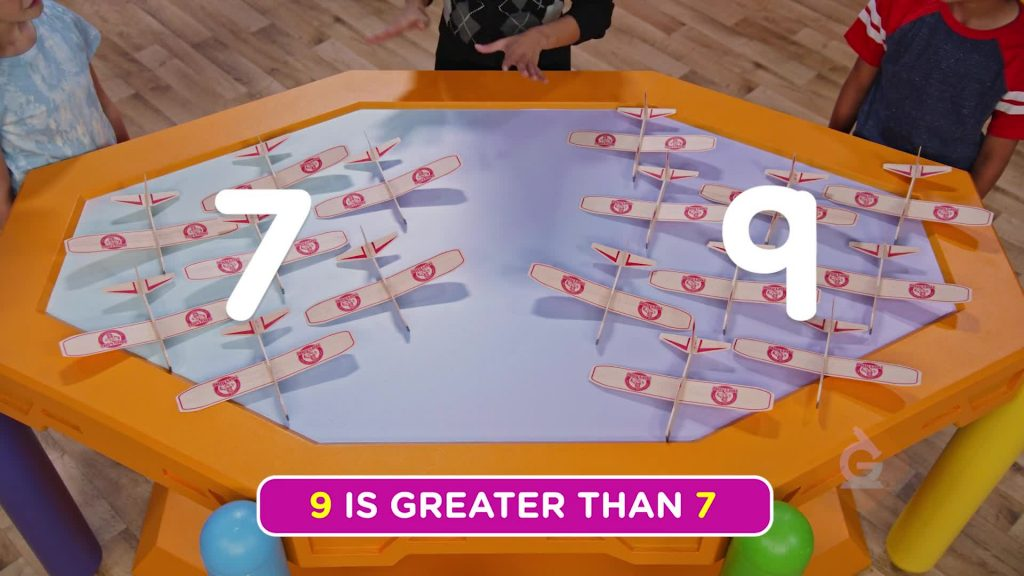WHAT IS COMPARING?

Comparing is when you find out which group has more things. You can compare by counting up the total amount in each group. The amount in the group can be greater than, less than or equal to the amount of things in another group.

To better understand comparing quantities up to 10…

WHAT IS COMPARING?. Comparing is when you find out which group has more things. You can compare by counting up the total amount in each group. The amount in the group can be greater than, less than or equal to the amount of things in another group. To better understand comparing quantities up to 10…

## LET’S BREAK IT DOWN!

### Compare 2 kinds of toys.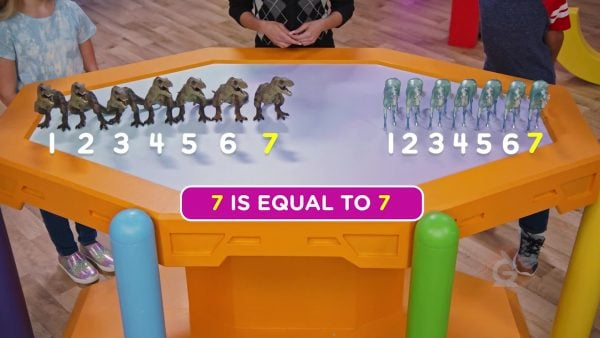Here we have 7 dinosaurs and 7 unicorns. You can show each amount using counters. 1, 2, 3, 4, 5, 6, 7. To compare these amounts, match dinosaurs and unicorns next to each other. Since these amounts are the same, we say they are equal.

Compare 2 kinds of toys. Here we have 7 dinosaurs and 7 unicorns. You can show each amount using counters. 1, 2, 3, 4, 5, 6, 7. To compare these amounts, match dinosaurs and unicorns next to each other. Since these amounts are the same, we say they are equal.

### Compare how many mice you and your friends have.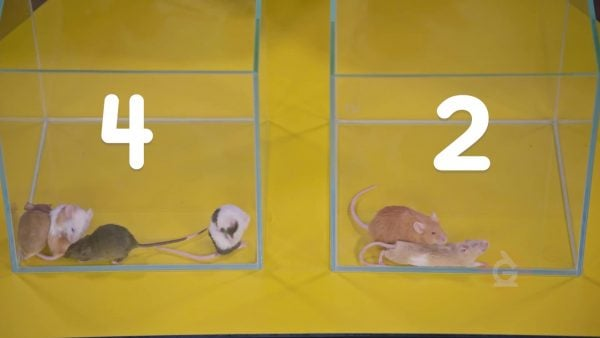You have 4 mice and your friend Erika has 2 mice. How can you compare these amounts? You can match them up next to each other, or you can notice that 4 comes after 2. This means that 4 is greater than 2, so you have more mice.

Compare how many mice you and your friends have. You have 4 mice and your friend Erika has 2 mice. How can you compare these amounts? You can match them up next to each other, or you can notice that 4 comes after 2. This means that 4 is greater than 2, so you have more mice.

### Compare how many toys you have.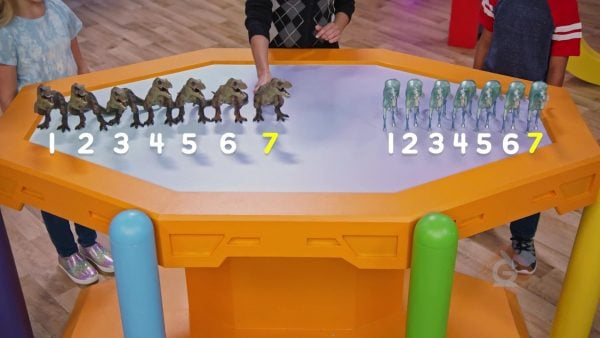You have 6 dinosaurs. You have 7 unicorns. How can you compare these amounts? You count past 6 to count to 7. 6 is less than 7. 7 is greater than 6.

Compare how many toys you have. You have 6 dinosaurs. You have 7 unicorns. How can you compare these amounts? You count past 6 to count to 7. 6 is less than 7. 7 is greater than 6.

### Compare how many planes there are.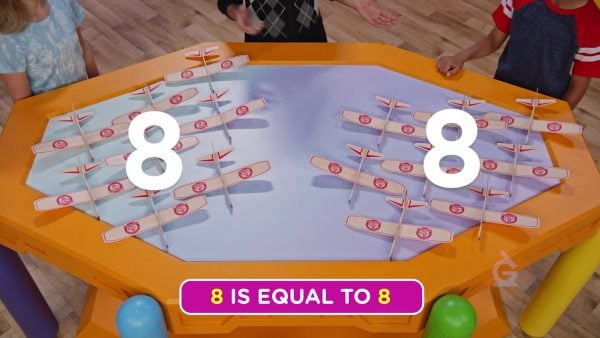Madelyn has 8 planes. Omar has 8 planes. 8 is equal to 8. They each have the same amount of planes to throw.

Compare how many planes there are. Madelyn has 8 planes. Omar has 8 planes. 8 is equal to 8. They each have the same amount of planes to throw.

### Compare with numbers without objects.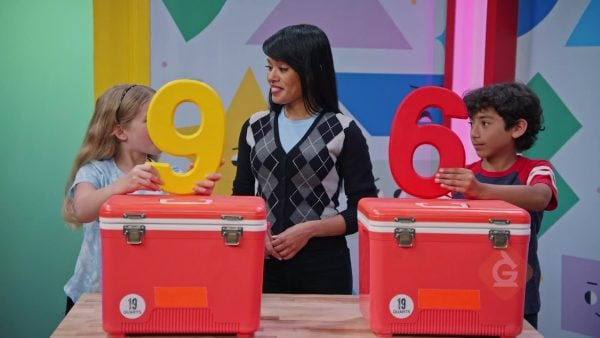You see the numbers 6 and 9. How can you compare 6 and 9? Count to 9: 1, 2, 3, 4, 5, 6, 7, 8, 9. You say 6 when counting to 9. 6 is less than 9.

Compare with numbers without objects. You see the numbers 6 and 9. How can you compare 6 and 9? Count to 9: 1, 2, 3, 4, 5, 6, 7, 8, 9. You say 6 when counting to 9. 6 is less than 9.

## COMPARING QUANTITIES UP TO 10 VOCABULARY

Greater than
When comparing two numbers, if the value of one number is more than the other, we say it is greater than.
Less than
When comparing two numbers, if the value of one number is smaller than the other, it is less than.
Equal
When comparing two numbers, if the values are the same, we say they are equal.
Compare
When comparing two numbers, determine if one number is greater than, less than or equal to the other number.
Count
When you count objects, you are finding how many you have in all.
Numbers
Symbols used to represent how many.

## COMPARING QUANTITIES UP TO 10 DISCUSSION QUESTIONS

### Model a group of 5 and a group of 3. Ask which group has a greater number of objects.

5 is greater than 3.

### Model a group of 6 and a group of 10. Ask which group has a lesser number of objects.

6 is less than 10.

Dane.

Six.

Two.
X

## Success

We’ve sent you an email with instructions how to reset your password.
Ok